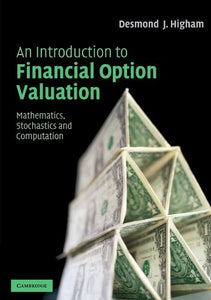# An Introduction To Financial Option Valuation: Mathematics, Stochastics And Computation

• Publish Date: 2004-04-19
• Binding: Paperback
• Author: Desmond J. Higham
Regular price \$85.35 Sale price \$29.61

Attention: For textbook, access codes and supplements are not guaranteed with used items.

This is a lively textbook providing a solid introduction to financial option valuation for undergraduate students armed with a working knowledge of a first year calculus. Written in a series of short chapters, its self-contained treatment gives equal weight to applied mathematics, stochastics and computational algorithms. No prior background in probability, statistics or numerical analysis is required. Detailed derivations of both the basic asset price model and the Black-Scholes equation are provided along with a presentation of appropriate computational techniques including binomial, finite differences and in particular, variance reduction techniques for the Monte Carlo method. Each chapter comes complete with accompanying stand-alone MATLAB code listing to illustrate a key idea. Furthermore, the author has made heavy use of figures and examples, and has included computations based on real stock market data.